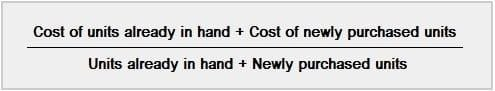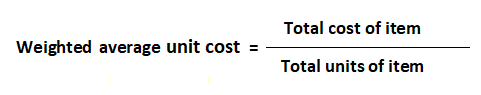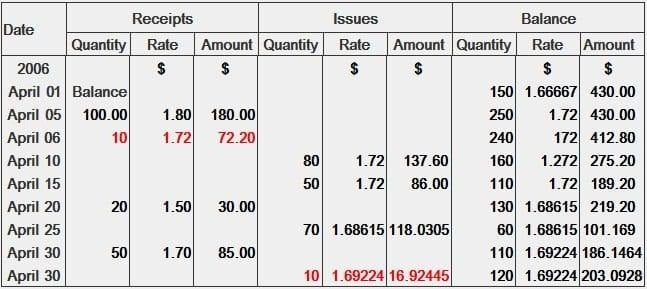# Weighted Average Method of Material Costing### Written byTrue Tamplin, BSc, CEPF®

Updated on March 29, 2022

## Weighted Average Cost: Definition

In the weighted average cost method, the average cost of materials purchased is charged to the job or process rather than the actual cost.

In other words, it is assumed that where a material is purchased at different prices, the unit cost will be the average cost of all units purchased over a particular period.

## Weighted Average Cost: Explanation

The weighted average cost method follows the concept of total stock and total valuation. This method of material costing is used for costing materials requisition and charging the cost of materials to production.

The balance on hand is also composed of units valued at the weighted average cost.

For example, suppose 50 units of Material X are purchased at \$10 per unit. Suppose that, a month later, another 50 units of Material X are purchased at \$12 per unit.

The cost to be charged to the job, process, department for Material X is \$11 per unit (i.e., the average cost).

To find the average price, the total cost of all materials of a particular class is divided by the number of units in hand.

When new materials are purchased, the average price is calculated as follows:

1. Add the quantity of units purchased to the quantity of units on hand
2. Add the cost of newly purchased materials to the cost of materials on hand
3. Divide the total cost (Step 2) by the total quantity (Step 1)

## Formula for Weighted Average Cost

One formula for weighted average cost is given as follows:Another version of this formula is shown below:When using both of the above formulas, students are advised to calculate average cost and round to five decimal places.

Materials are issued from the store at the established average cost until a new purchase is recorded.

Although a new average price may be computed when materials are returned to sellers and when excess issues are returned to the store, it seems sufficient for practical purposes to reduce or increase the total quantity and cost, allowing the unit price to remain unchanged.

This means that materials returned to sellers and to the store are recorded at the present average cost.

## Advantage of Weighted Average Cost Method

The main advantages of the weighted average costing method include:

• Minimizes the effect of unusually high and low material prices
• Practical and suitable for charging the cost of materials used to production
• Enables management to analyze operating results
• Simple to apply when receipts for materials are not numerous

## Disadvantages of Weighted Average Cost Method

The main disadvantages of the weighted average costing method include:

• Materials used may not be charged to production at the current price
• The cost charged to production is not the actual cost
• If receipts are numerous, many calculations are required

## Example

Consider the following information:

• April 01: Inventories on hand are 50 units at the rate of \$2 and 100 units at the rate of \$4.50
• April 05: 100 units purchased at \$1.80
• April 06: 10 units of the inventories purchased on 5 April at the rate of \$1.80 are returned to supplier
• April 10: 80 units issued to factory
• April 15: 50 units issued to factory
• April 20: 20 units purchased at the rate of \$1.50
• April 25: 70 units issued to factory
• April 30: 50 units purchased at \$1.70

Determine the cost of inventory on 30 April using the weighted average method of costing.

### Solution## Weighted Average Method of Material Costing FAQs